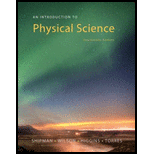# Which combination of units expresses density? (a) mass/(time) 3 (b) mass/(kg) 3 (c) mass/(length) 3 (d) mass/m 2### An Introduction to Physical Science

14th Edition
James Shipman + 3 others
Publisher: Cengage Learning
ISBN: 9781305079137### An Introduction to Physical Science

14th Edition
James Shipman + 3 others
Publisher: Cengage Learning
ISBN: 9781305079137

#### Solutions

Chapter
Section
Chapter 1, Problem 10MC
Textbook Problem
535 views

## Which combination of units expresses density? (a) mass/(time)3 (b) mass/(kg)3 (c) mass/(length)3 (d) mass/m2

Expert Solution
To determine
Unit of density.

### Explanation of Solution

Given Info: Unit of density is given.

Explanation:

A derived unit is combination and multiples of standard unit. For example density,

ρ=mV

Here,

m is the mass

V is the volume

Unit of mass is kilogram (kg) and unit of volume is cubic centimeter (cm3) .

Derived unit of density is,

ρ=m(kg)V(

### Want to see the full answer?

Check out a sample textbook solution.See solution

### Want to see this answer and more?

Bartleby provides explanations to thousands of textbook problems written by our experts, many with advanced degrees!

See solution